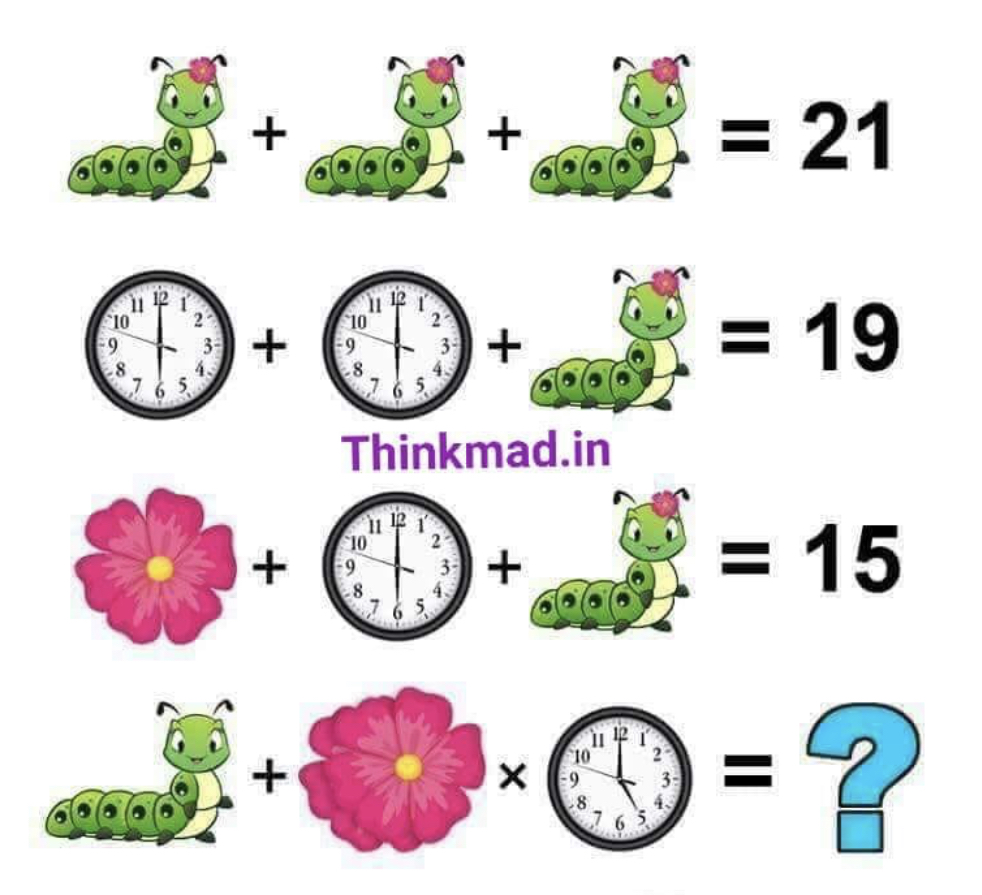# the caterpillar, clock and flower puzzle### Help Support UKworkshop.co.uk:#### RobinBHM

##### Established Member
There is more to it than an initial glance would suggest.......
(study each item carefully)66

#### MikeG.

##### Damocles.
25

A: (Caterpillar +Flower) x 3 = 21.....therefore (cat + fl) = 7

B: (6 O'clock) + (6 O'clock) + (cat + fl) = 19
(6 O'clock) + (6 O'clock) + 7 = 19
6 O'Clock = 6

C: Flower + (6 O'clock) + (cat + flower) = 15
flower + (6) + (7) = 15
therefore flower = 15-13=2

From A
If cat + flower = 7, and flower = 2 (from C), then caterpillar = 5

So D....

Cat + 2 flowers x 5 Oclock = ??
5 + 2(2) x 5 (assuming hours = value) = ??
Remembering order of arithmetical functions...

5 + (4 x 5) = 25

#### Droogs

##### Is that chisel shar ... Ow
none of the shown elements in the last problem are known and can not be worked out using the previous examples

#### nev

##### Established Member
in my old school it would be 45

#### D_W

##### Established Member
If this were a computer programming language, you would be forced to define the order of operations.

We use two software packages at work and they each would calculate the result differently (parens work to set priority in both and are mandatory as mistakes are not tolerable).

#### Trainee neophyte

##### [Insert witty and amusing title here]
As the arithmetic answer is already shown, I will go with the answer being "gin and tonic"

(It's always 5 o'clock somewhere)

#### Droogs

##### Is that chisel shar ... Ow
MikeG.":151vo9jm said:
25

A: (Caterpillar +Flower) x 3 = 21.....therefore (cat + fl) = 7

B: (6 O'clock) + (6 O'clock) + (cat + fl) = 19
(6 O'clock) + (6 O'clock) + 7 = 19
6 O'Clock = 6

C: Flower + (6 O'clock) + (cat + flower) = 15
flower + (6) + (7) = 15
therefore flower = 15-13=2

From A
If cat + flower = 7, and flower = 2 (from C), then caterpillar = 5

So D....

Cat + 2 flowers x 5 Oclock = ??
5 + 2(2) x 5 (assuming hours = value) = ??
Remembering order of arithmetical functions...

5 + (4 x 5) = 25
But the caterpillar in the bottom example is a completely different type of caterpillar - not only is it minus a flower it has an extra body segment and a higher number of legs so can not be taken to be cat with flower sans flower as not the same.
asuming that clack time equals value introduces an uncertaincy to the equation and is a no no

Therefore insufficient information to solve last puzzle - oh and a flower with one behind it does in no way mean that it is equal to 2 x value of flower

#### Stanleymonkey

##### Established Member
Sneaky double flower at the end

Most people would read left to right and make 66

Multiplying first would make 31?

#### Stanleymonkey

##### Established Member
Just spotted the flower on the head! Doh! and the clock change. Going for a lie down

#### MikeG.

##### Damocles.
Droogs":2pztunnj said:
......... the caterpillar in the bottom example is a completely different type of caterpillar........it has an extra body segment and a higher number of leg
I missed that in my rush to do the arithmetic.

that clack time equals value introduces an uncertaincy to the equation and is a no no
My assumption was that there was a solution, so I looked for the pattern. We are, after all, pattern-seekers.

Therefore insufficient information to solve last puzzle - oh and a flower with one behind it does in no way mean that it is equal to 2 x value of flower
I agree. My maths was right, but my underlying assumptions were wrong. There are too many unknowns and assumptions to be be certain of the answer. These internet maths/ logic arguments always boil down to ambiguity. It's their raison d'etre.

#### Droogs

##### Is that chisel shar ... Ow
Only noticed as i play a lot of mahjong and used to be an air cadet aircraft recognition competition competitor where the difference in aircraft type might just be the shape or placement of refueling probe or even an access panel.

#### D_W

##### Established Member
Seeing the caterpillar trick, I call this age discrimination.

The older we are, the more we're likely to make assumptions working through something.

#### RogerS

##### Established Member
Droogs":1xhrtog2 said:
Only noticed as i play a lot of mahjong and used to be an air cadet aircraft recognition competition competitor where the difference in aircraft type might just be the shape or placement of refueling probe or even an access panel.
Ah...commeth the hour...commeth the man.

Is this a Sukhoi or a MIG ?I can't make up my mind#### Attachments

• 1.2 MB Views: 363

#### Bm101

##### Lean into the curve.
50 (homer)
Standing on the shoulders of giants.

#### heronviewer

##### Established Member
caterpillar = no of segments
flower = 2
caterpillar + flower = no of segments + 2
clock = time showing

#### MikeG.

##### Damocles.
6 + 4 x 5 does not equal 50, people. It equals 26.

(6+4)x5=50 !

#### MikeG.

##### Damocles.
Where do those brackets come from? They're not there in the original.